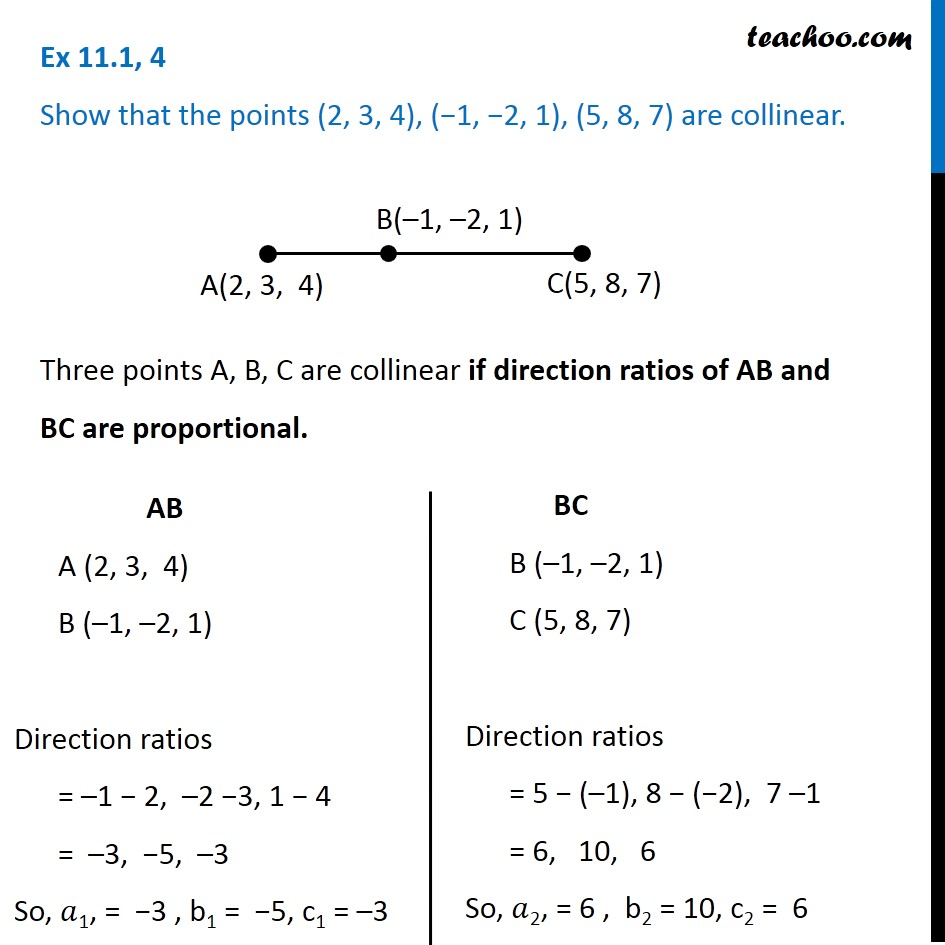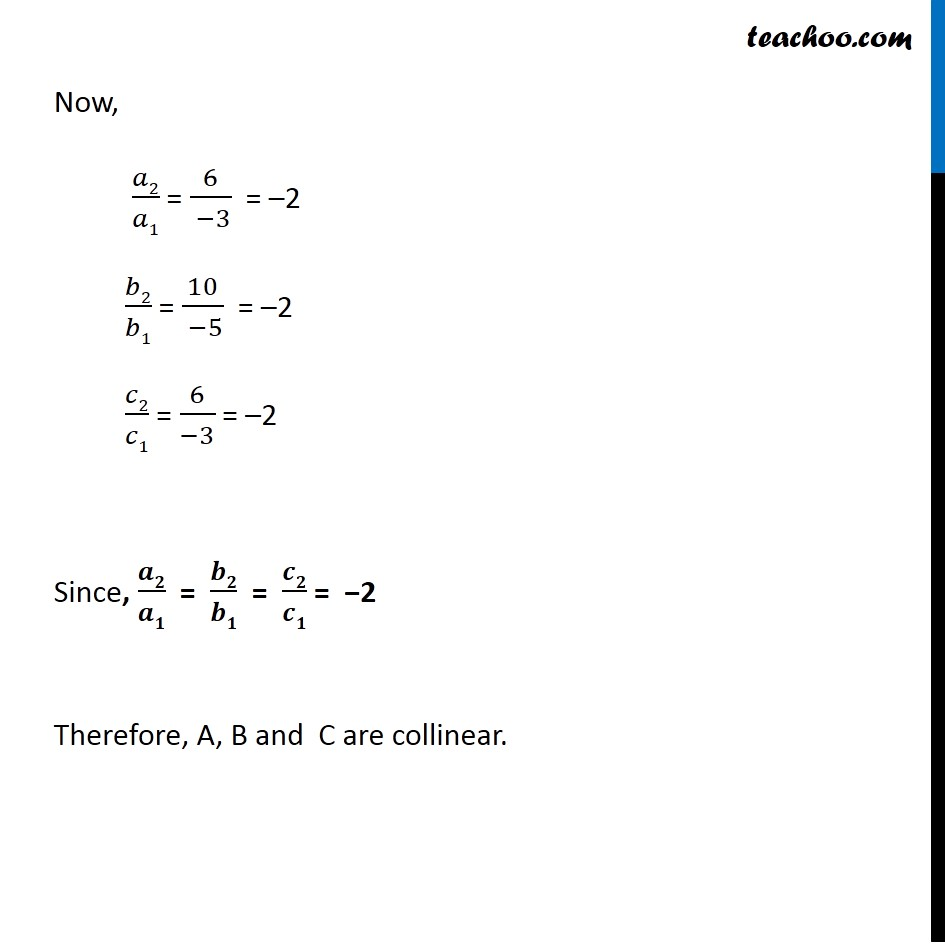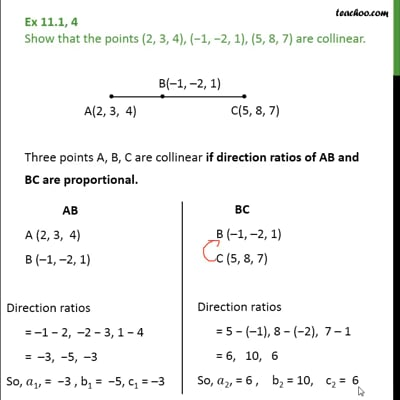Ex 11.1

Chapter 11 Class 12 Three Dimensional Geometry
Serial order wiseThis video is only available for Teachoo black users

Solve all your doubts with Teachoo Black (new monthly pack available now!)

### Transcript

Ex 11.1, 4 Show that the points (2, 3, 4), (−1, −2, 1), (5, 8, 7) are collinear. Three points A, B, C are collinear if direction ratios of AB and BC are proportional. AB A (2, 3, 4) B (–1, –2, 1) Direction ratios = –1 − 2, –2 −3, 1 − 4 = –3, −5, –3 So, 𝑎1, = −3 , b1 = −5, c1 = –3 BC B (–1, –2, 1) C (5, 8, 7) Direction ratios = 5 − (–1), 8 − (−2), 7 –1 = 6, 10, 6 So, 𝑎2, = 6 , b2 = 10, c2 = 6 Now, 𝑎2/𝑎1 = 6/( −3) = –2 𝑏2/𝑏1 = 10/( −5) = –2 𝑐2/𝑐1 = 6/(−3) = –2 Since, 𝒂𝟐/𝒂𝟏 = 𝒃𝟐/𝒃𝟏 = 𝒄𝟐/𝒄𝟏 = −2 Therefore, A, B and C are collinear.